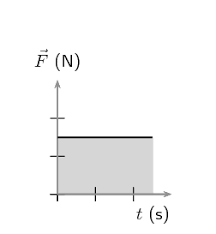## How to Calculate and Solve for Impulse | MotionThe image above represents impulse.

To compute for impulse, two essential parameters are needed and these parameters are force (f) and time (t).

The formula for calculating impulse:

I = Ft

Where;

I = Impulse
F = Force
t = Time

Let’s solve an example;
Given that the force is 28 and the time is 14. Find the impulse?

This implies that;

F = Force = 28
t = Time = 14

I = Ft
I = 28 x 14
I = 392

Therefore, the impulse is 392 Ns.

Calculating the Force when the Impulse and the Time is Given.

F = I / t

Where;

F = Force
I = Impulse
t = Time

Let’s solve an example;
Given that the impulse is 60 and the time is 10. Find the force?

This implies that;

I = Impulse = 60
t = Time = 10

F = I / t
F = 60 / 10
F = 6

Therefore, the force is 6 N.